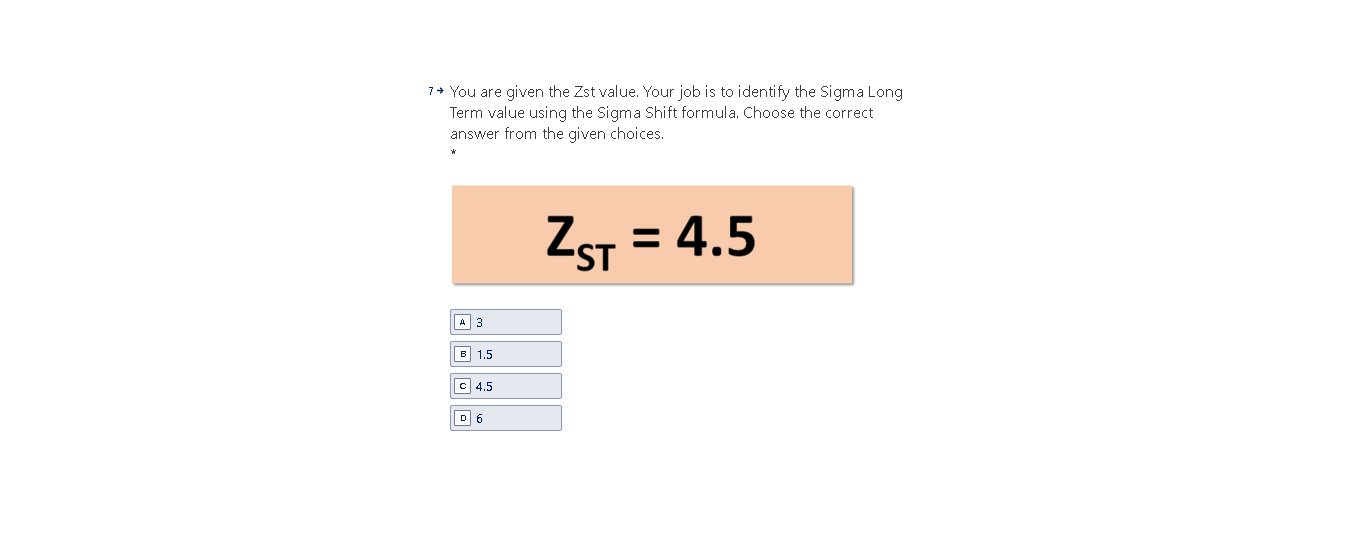# Question Solved1 Answer7 + You are given the Zst value. Your job is to identify the Sigma Long Term value using the Sigma Shift formula. Choose the correct answer from the given choices. 2 ST = 4.5 A 3 B 1.5 C 4.5 D 6BM1T9O The Asker · Probability and StatisticsTranscribed Image Text: 7 + You are given the Zst value. Your job is to identify the Sigma Long Term value using the Sigma Shift formula. Choose the correct answer from the given choices. 2 ST = 4.5 A 3 B 1.5 C 4.5 D 6
More
Transcribed Image Text: 7 + You are given the Zst value. Your job is to identify the Sigma Long Term value using the Sigma Shift formula. Choose the correct answer from the given choices. 2 ST = 4.5 A 3 B 1.5 C 4.5 D 6